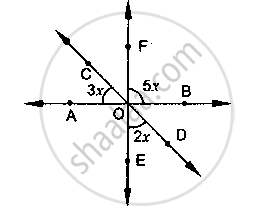# In the Below Fig, Find the Value of X. - Mathematics

In the below fig, find the value of x.#### Solution

Vertically opposite angles are equal

∠AOE = ∠BOF = 5x

Linear pair

∠COA + ∠AOE + ∠EOD = 180°

⇒ 3x + 5x + 2x = 180°

⇒ 10x = 180°

⇒ x = 18°

Concept: Pairs of Angles
Is there an error in this question or solution?

#### APPEARS IN

RD Sharma Mathematics for Class 9
Chapter 10 Lines and Angles
Exercise 10.3 | Q 4 | Page 22